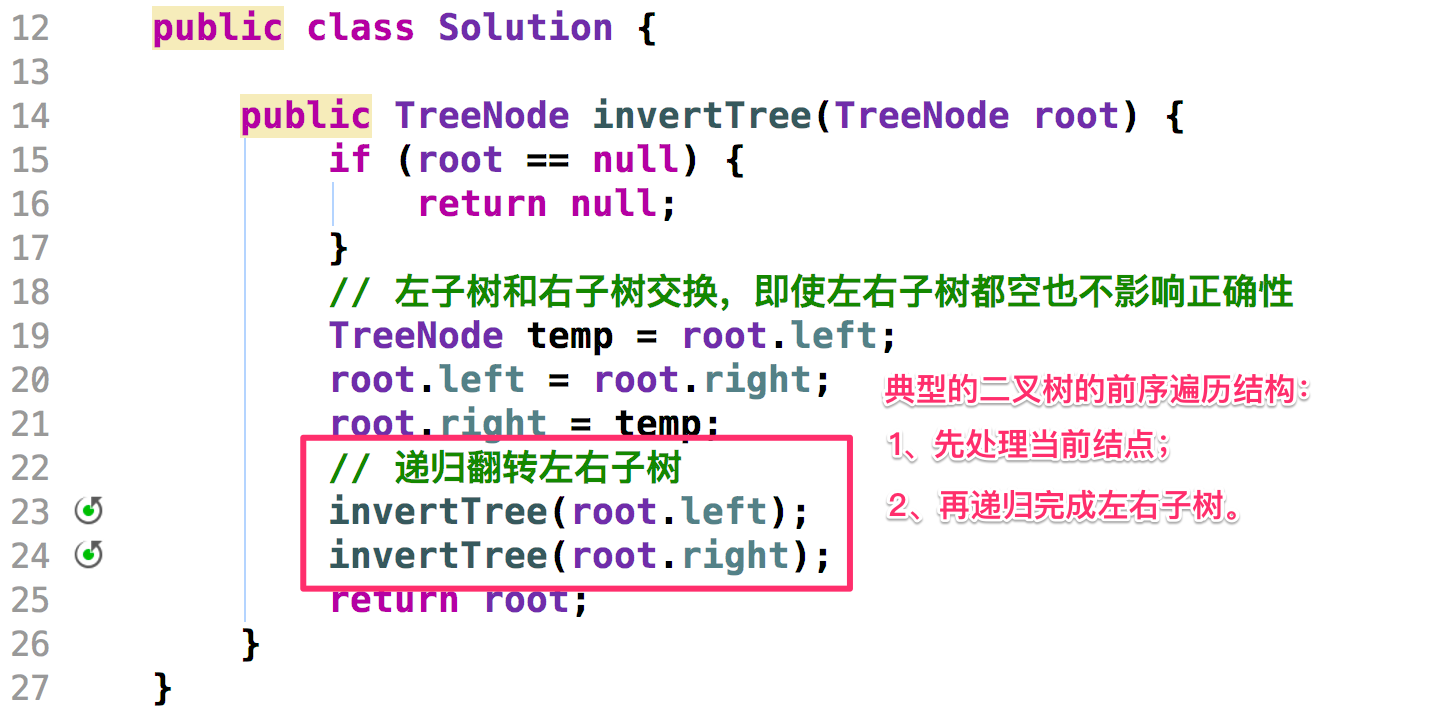# 「二叉树」专题 2：反转一棵二叉树

## 「二叉树」专题 2：反转一棵二叉树

### 「力扣」第 226 题：反转一棵二叉树

4
/   \
2     7
/ \   / \
1   3 6   9

4
/   \
7     2
/ \   / \
9   6 3   1

Java 代码：Java 代码：后序遍历

class TreeNode {
int val;
TreeNode left;
TreeNode right;

TreeNode(int x) {
val = x;
}
}

public class Solution {
public TreeNode invertTree(TreeNode root) {
if (root == null) {
return null;
}
invertTree(root.left);
invertTree(root.right);
// swap root.left 和  root.right
TreeNode temp = root.left;
root.left = root.right;
root.right = temp;
return root;
}
}

Java 代码：与上面的代码等价

public class Solution2 {

public TreeNode invertTree(TreeNode root) {
if (root == null) {
return root;
}
TreeNode left = root.left;
TreeNode right = root.right;
root.left = invertTree(right);
root.right = invertTree(left);
return root;
}
}

### 方法一：前序遍历

Java 代码：

class TreeNode {
int val;
TreeNode left;
TreeNode right;

TreeNode(int x) {
val = x;
}
}

public class Solution {

public TreeNode invertTree(TreeNode root) {
if (root == null) {
return null;
}

// 左子树和右子树交换，即使左右子树都空也不影响正确性
TreeNode temp = root.left;
root.left = root.right;
root.right = temp;

// 递归翻转左右子树
invertTree(root.left);
invertTree(root.right);
return root;
}
}

### 方法二：中序遍历

Java 代码：

public class Solution {

public TreeNode invertTree(TreeNode root) {
if (root == null) {
return null;
}

invertTree(root.left);

TreeNode temp = root.left;
root.left = root.right;
root.right = temp;

// 注意：因为左右子树已经交换了，因此这里不能写 invertTree(root.right);
invertTree(root.left);
return root;
}
}


### 方法三：后序遍历

Java 代码：

public class Solution {

public TreeNode invertTree(TreeNode root) {
if (root == null) {
return null;
}

invertTree(root.left);
invertTree(root.right);

TreeNode temp = root.left;
root.left = root.right;
root.right = temp;
return root;
}
}

### 方法四：层序遍历

Java 代码：

import java.util.LinkedList;
import java.util.Queue;

public class Solution {

public TreeNode invertTree(TreeNode root) {
// 结点为空的特殊情况要先考虑
if (root == null) {
return null;
}

queue.offer(root);
while (!queue.isEmpty()) {
TreeNode curNode = queue.poll();
// 只要其中之一非空，我都交换，并且把非空的结点添加到队列里
if (curNode.left != null || curNode.right != null) {
// 先翻转
TreeNode temp = curNode.left;
curNode.left = curNode.right;
curNode.right = temp;
// 把非空的节点加入队列
if (curNode.left != null) {
queue.offer(curNode.left);
}
if (curNode.right != null) {
queue.offer(curNode.right);
}
}
}
return root;
}
}上一篇「树」专题 1：树与递归
「树」专题 1：树与递归 树是理解「递归」和「分治」算法的很好的数据结构； 很多高级数据结构都是从「树」来的。 我们开始介绍「二叉树和递归」。递归，是使用计算机解决问题的一种重要的思考方式。而二叉树由于其天然的递归结构，使得基于二叉树的算
2017-11-16
目录